# Test: Geometry- 1

## 15 Questions MCQ Test IBPS PO Prelims- Study Material, Online Tests, Previous Year | Test: Geometry- 1

Description
Attempt Test: Geometry- 1 | 15 questions in 30 minutes | Mock test for UPSC preparation | Free important questions MCQ to study IBPS PO Prelims- Study Material, Online Tests, Previous Year for UPSC Exam | Download free PDF with solutions
QUESTION: 1

### A vertical stick 20 m long casts a shadow 10 m long on the ground. At the same time, a tower casts a shadow 50 m long on the ground. Find the height of the tower.

Solution:
• When the length of stick = 20 m, then length of shadow = 10 m i.e. in this case length = 2 * shadow
• With the same angle of inclination of the sun, the length of the tower that casts a shadow of 50 m is: 2 * 50m = 100m
⇒ Height of tower = 100 m
QUESTION: 2

### The area of similar triangles, ABC and DEF are 144cm2 and 81 cm2 respectively. If the longest side of the larger △ABC be 36 cm, then the longest side of the smaller △DEF is:

Solution:

For similar triangles

⇒ (Ratio of sides) = Ratio of areas

Then as per question = (36/x)= 141/81

Let the longest side of ΔDEF = x

⇒ 36/x = 12/9

⇒ x = 27cm

QUESTION: 3

### Find the value of x in the figure, if it is given that AC and BD are diameters of the circle.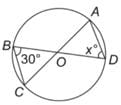Solution:
• The triangle BOC is an isosceles triangle with sides OB and OC both being equal as they are the radii of the circle. Hence, the angle OBC = angle OCB = 30°.
• Hence, the third angle of the triangle BOC i.e. Angle BOC would be equal to 120°.
⇒ BOC = AOD = 120°
• Also, in the isosceles triangle DOA:
Angle ODA = Angle DAO = x = 30°
QUESTION: 4

Find the value of x in the given figure.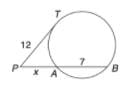Solution:

By the rule of tangents, we get:

⇒ 122 = (x + 7)x
⇒ 144 = x2 + 7x
⇒ x2 + 7x – 144 = 0
⇒ x2 +16x – 9x –144 = 0
⇒ x(x + 16) – 9(x + 16) = 0
⇒ x = 9 or –16

–16 can’t be the length, hence this value is discarded. Thus, x = 9

QUESTION: 5

Find the value of x in the given figure.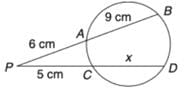Solution:

By the rule of chords, cutting externally, we get:

(9 + 6) * 6 = (5 + x) * 5
90 = 25 + 5x
5x = 65
x = 13 cm

QUESTION: 6

In the given figure ㄥPAB = 25º. AB is the diameter. Find ㄥTPA.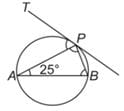Solution:
• ㄥAPB = 90° (angle in a semicircle = 90°)
• ㄥPBA = 180 – (90 + 25) = 65°
• ㄥTPA = ㄥPBA (the angle that a chord makes with the tangent, is subtended by the chord on the circumference in the alternate segment) = 65°

Note: This is also called the Alternate Segment Theorem.

QUESTION: 7

In the figure, AB is parallel to CD and RD || SL || TM || AN, and BR : RS : ST : TA = 3 : 5 : 2 : 7. If it is known that CN = 1.333 BR. Find the ratio of BF : FG : GH : HI : IC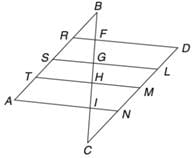Solution:
• Since the lines, AB and CD are parallel to each other, and the lines RD and AN are parallel, it means that the triangles RBF and NCI are similar to each other. Since the ratio of CN : BR = 1.333, if we take BR as 3, we will get CN as 4.
• This means that the ratio of BF : CI would also be 3 : 4.
Also, the ratio of BR : RS : ST : TA = BF : FG : GH : HI = 3 : 5 : 2 : 7 (given).

Hence, the correct answer is 3 : 5 : 2 : 7 : 4

QUESTION: 8

In the following figure, it is given that O is the centre of the circle and ㄥAOC = 140°. Find ㄥABC.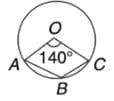Solution: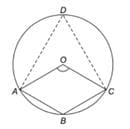∠AOC of minor sector = 140°
∠AOC of major sector=360° - 140° = 220°
Theorem: The angle subtended at the centre is twice the angle formed at the circumference of the circle.
Hence,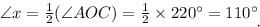∴ The measure of ∠x = 110°

QUESTION: 9

In the figure below, PQ = QS, QR = RS and angle SRQ = 100°. How many degrees is angle QPS?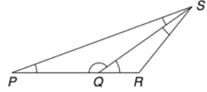Solution:

In ΔQRS, QR = RS
⇒ ㄥRQS = ㄥRSQ (because angles opposite to equal sides are equal).
Thus:

ㄥRQS + ㄥRSQ = 180° - 100° = 80°
ㄥRQS = ㄥRSQ = 40°
ㄥPQS = 180° – 40° = 140°  (sum of angles on a line = 180°)

Then again, ㄥQPS = ㄥQSP (since angles opposite to equal sides are equal)

ㄥQPS + ㄥQSP = 180° – 140° = 40°
ㄥQPS = ㄥQSP = 20°

QUESTION: 10

In the given figure, AD is the bisector of ΔBAC, AB = 6 cm, AC = 5 cm and BD = 3 cm. Find DC. It is given that ∠ABD = ∠ACD.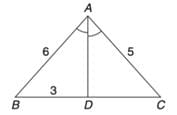Solution:

We know that the internal bisector of angle of a triangle divides the opposite side internally in the ratio of the sides containing the angle.
Hence:

AB/BD = AC/CD
6/3 = 5/CD
CD = 2.5 cm

QUESTION: 11

In the given figure, straight line AB and CD intersect at O. IF ∠δ =3∠v, then ∠v = ?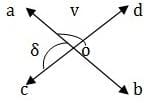Solution:

COD is a  straight line
∴ ∠δ + ∠v =180⁰ ⇒ 3v +v =180 ⇒ 4v = 180 ⇒ v =45⁰.

QUESTION: 12

In a triangle ABC, the incentre is at 0. If ㄥBOC = 100°, find ㄥBAC.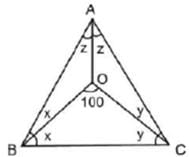Solution:

In ∠BOC

⇒ x + y = 80°

⇒ 2x + 2y. = 160°

Also, 2x + 2y + 2z = 180°

⇒ 160° + 2z = 180°

⇒ ∠BAC = 2z = 20°

QUESTION: 13

A rectangular enclosure 40 m x 36 m has a horse tethered to a corner with a rope of 14 m in length. What is the ratio of the respective areas it can graze if it is outside the enclosure and if it is inside the enclosure?

Solution: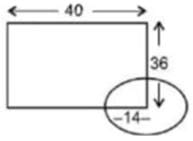• Imagine a circle on the corner of the rectangle
• 3 quarters of the circle lie outside the rectangle and 1 quarter lies inside.
• Hence, Required ratio = 3 : 1
QUESTION: 14

Read the passage below and solve the questions based on it.
The area of a square is equal to the area of a rectangle. Moreover, the perimeter of the square is also equal to the perimeter of the rectangle.

Q. The length of the rectangle is equal to the:

Solution:

This is possible only when both the length and breadth of the rectangle are equal to the side of the square.

QUESTION: 15

A quadrilateral is inscribed in a circle. If an angle is inscribed in each of the four segments outside the quadrilateral, then what is the sum of these four angles?

Solution: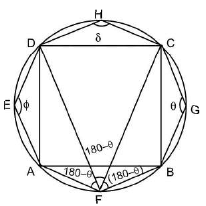Required sum = θ + 180° - θ + 180° - θ + 180° - θ + θ + 8 = 540°Use Code STAYHOME200 and get INR 200 additional OFF Use Coupon Code